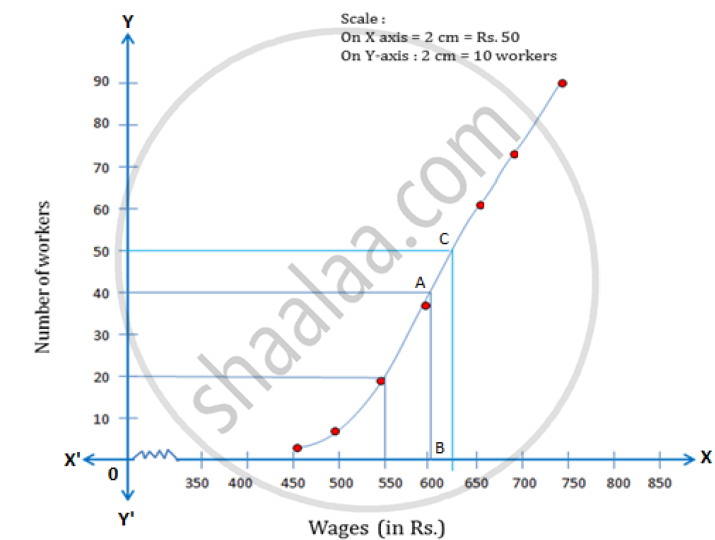Share

# The Daily Wages of 80 Workers in a Project Are Given Below. Use a Graph Paper to Draw an Ogive for the Above Distribution. (Use a Scale of 2 Cm = Rs. 50 on X-axis and 2 Cm = 10 Workers on Y-axis). Use Your Ogive to Estimate - ICSE Class 10 - Mathematics

ConceptOgives (Cumulative Frequency Graphs)

#### Question

The daily wages of 80 workers in a project are given below.

 Wages(in Rs.) 400-450 450-500 500-550 550-600 600-650 650-700 700-750 No. ofWorkers 2 6 12 18 24 13 5

Use a graph paper to draw an ogive for the above distribution. (Use a scale of 2 cm = Rs.
50 on x-axis and 2 cm = 10 workers on y-axis). Use your ogive to estimate:

1) the median wage of the workers

2) the lower quartile wage of workers

3) the numbers of workers who earn more than Rs. 625 daily

#### Solution

The cumulative frequency table of the given distribution is as follows:

 Wages in Rs Upper Limit No. of workers Cumulative frequency 400-450 450 2 2 450-500 500 6 8 500-550 550 12 20 550-600 600 18 38 600-650 650 24 62 650-700 700 13 75 700-750 750 5 80

The ogive is as follows:Number of workers = n = 80

1) Median = (n/2)th term = 40th term

Through mark 40 on the Y-axis, draw a horizontal line which meets the curve at point A.

Through point A, on the curve draw a vertical line which meets the X-axis at point B

The value of point B on the X-axis is the median, which is 605.

2) Lower quartile (Q_1) = (80/4)th term = 20th term = 550

3) Through mark of 625 on X-axis, draw a verticle line which meets the graph at point C.

Then through point C, draw a horizontal line which meets the Y-axis at the mark of 50.

Thus, the number of workers that earn more than Rs. 625 daily =  80-50 = 30

Is there an error in this question or solution?

#### Video TutorialsVIEW ALL 

Solution The Daily Wages of 80 Workers in a Project Are Given Below. Use a Graph Paper to Draw an Ogive for the Above Distribution. (Use a Scale of 2 Cm = Rs. 50 on X-axis and 2 Cm = 10 Workers on Y-axis). Use Your Ogive to Estimate Concept: Ogives (Cumulative Frequency Graphs).
S